This document was generated on CPALMS - www.cpalms.org
You are not viewing the current course, please click the current year’s tab.

#### Course Standards

Name Description
MA.3.AR.1.1: Apply the distributive property to multiply a one-digit number and two-digit number. Apply properties of multiplication to find a product of one-digit whole numbers.
 Clarifications:Clarification 1: Within this benchmark, the expectation is to apply the associative and commutative properties of multiplication, the distributive property and name the properties. Refer to K-12 Glossary (Appendix C). Clarification 2: Within the benchmark, the expectation is to utilize parentheses. Clarification 3: Multiplication for products of three or more numbers is limited to factors within 12. Refer to Properties of Operations, Equality and Inequality (Appendix D).

 Examples:The product 4×72 can be found by rewriting the expression as 4×(70+2) and then using the distributive property to obtain (4×70)+(4×2) which is equivalent to 288.
MA.3.AR.1.2: Solve one- and two-step real-world problems involving any of four operations with whole numbers.
 Clarifications:Clarification 1: Instruction includes understanding the context of the problem, as well as the quantities within the problem. Clarification 2: Multiplication is limited to factors within 12 and related division facts. Refer to Situations Involving Operations with Numbers (Appendix A).

 Examples:A group of students are playing soccer during lunch. How many students are needed to form four teams with eleven players each and to have two referees?
MA.3.AR.2.1: Restate a division problem as a missing factor problem using the relationship between multiplication and division.
 Clarifications:Clarification 1: Multiplication is limited to factors within 12 and related division facts. Clarification 2: Within this benchmark, the symbolic representation of the missing factor uses any symbol or a letter.

 Examples:The equation 56÷7=? can be restated as 7×?=56 to determine the quotient is 8.
MA.3.AR.2.2: Determine and explain whether an equation involving multiplication or division is true or false.
 Clarifications:Clarification 1: Instruction extends the understanding of the meaning of the equal sign to multiplication and division. Clarification 2: Problem types are limited to an equation with three or four terms. The product or quotient can be on either side of the equal sign. Clarification 3: Multiplication is limited to factors within 12 and related division facts.

 Examples:Given the equation 27÷3=3×3 , it can be determined to be a true equation by dividing the numbers on the left side of the equal sign and multiplying the numbers on the right of the equal sign to see that both sides are equivalent to 9.
MA.3.AR.2.3: Determine the unknown whole number in a multiplication or division equation, relating three whole numbers, with the unknown in any position.
 Clarifications:Clarification 1: Instruction extends the development of algebraic thinking skills where the symbolic representation of the unknown uses any symbol or a letter. Clarification 2: Problems include the unknown on either side of the equal sign. Clarification 3: Multiplication is limited to factors within 12 and related division facts. Refer to Situations Involving Operations with Numbers (Appendix A).
MA.3.AR.3.1: Determine and explain whether a whole number from 1 to 1,000 is even or odd.
 Clarifications:Clarification 1: Instruction includes determining and explaining using place value and recognizing patterns.
MA.3.AR.3.2: Determine whether a whole number from 1 to 144 is a multiple of a given one-digit number.
 Clarifications:Clarification 1: Instruction includes determining if a number is a multiple of a given number by using multiplication or division.
MA.3.AR.3.3: Identify, create and extend numerical patterns.
 Clarifications:Clarification 1: The expectation is to use ordinal numbers (1st, 2nd, 3rd, …) to describe the position of a number within a sequence. Clarification 2: Problem types include patterns involving addition, subtraction, multiplication or division of whole numbers.

 Examples:Bailey collects 6 baseball cards every day. This generates the pattern 6,12,18,… How many baseball cards will Bailey have at the end of the sixth day?
MA.3.DP.1.1: Collect and represent numerical and categorical data with whole-number values using tables, scaled pictographs, scaled bar graphs or line plots. Use appropriate titles, labels and units.
 Clarifications:Clarification 1: Within this benchmark, the expectation is to complete a representation or construct a representation from a data set.  Clarification 2: Instruction includes the connection between multiplication and the number of data points represented by a bar in scaled bar graph or a scaled column in a pictograph.Clarification 3: Data displays are represented both horizontally and vertically.
MA.3.DP.1.2: Interpret data with whole-number values represented with tables, scaled pictographs, circle graphs, scaled bar graphs or line plots by solving one- and two-step problems.
 Clarifications:Clarification 1: Problems include the use of data in informal comparisons between two data sets in the same units.  Clarification 2: Data displays can be represented both horizontally and vertically. Clarification 3: Circle graphs are limited to showing the total values in each category.
MA.3.FR.1.1: Represent and interpret unit fractions in the form 1/n as the quantity formed by one part when a whole is partitioned into n equal parts.
 Clarifications:Clarification 1: This benchmark emphasizes conceptual understanding through the use of manipulatives or visual models. Clarification 2: Instruction focuses on representing a unit fraction as part of a whole, part of a set, a point on a number line, a visual model or in fractional notation. Clarification 3: Denominators are limited to 2, 3, 4, 5, 6, 8, 10 and 12.

 Examples: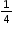can be represented as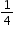of a pie (parts of a shape), as 1 out of 4 trees (parts of a set) or ason the number line.
MA.3.FR.1.2: Represent and interpret fractions, including fractions greater than one, in the form of $\frac{m}{n}$ as the result of adding the unit fraction $\frac{1}{n}$ to itself times.
 Clarifications:Clarification 1: Instruction emphasizes conceptual understanding through the use of manipulatives or visual models, including circle graphs, to represent fractions. Clarification 2: Denominators are limited to 2, 3, 4, 5, 6, 8, 10 and 12.

 Examples: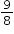can be represented as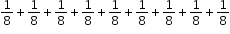.
MA.3.FR.1.3: Read and write fractions, including fractions greater than one, using standard form, numeral-word form and word form.
 Clarifications:Clarification 1: Instruction focuses on making connections to reading and writing numbers to develop the understanding that fractions are numbers and to support algebraic thinking in later grades. Clarification 2: Denominators are limited to 2, 3, 4, 5, 6, 8, 10 and 12.

 Examples:The fraction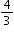written in word form is four-thirds and in numeral-word form is 4 thirds.
MA.3.FR.2.1: Plot, order and compare fractional numbers with the same numerator or the same denominator.
 Clarifications:Clarification 1: Instruction includes making connections between using a ruler and plotting and ordering fractions on a number line. Clarification 2: When comparing fractions, instruction includes an appropriately scaled number line and using reasoning about their size. Clarification 3: Fractions include fractions greater than one, including mixed numbers, with denominators limited to 2, 3, 4, 5, 6, 8, 10 and 12.

 Examples:The fraction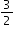is to the right of the fraction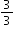on a number line sois greater than.
MA.3.FR.2.2: Identify equivalent fractions and explain why they are equivalent.
 Clarifications:Clarification 1: Instruction includes identifying equivalent fractions and explaining why they are equivalent using manipulatives, drawings, and number lines. Clarification 2: Within this benchmark, the expectation is not to generate equivalent fractions. Clarification 3: Fractions are limited to fractions less than or equal to one with denominators of 2, 3, 4, 5, 6, 8, 10 and 12. Number lines must be given and scaled appropriately.

 Examples:Example: The fractions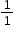andcan be identified as equivalent using number lines. Example: The fractions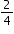and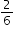can be identified as not equivalent using a visual model.
MA.3.GR.1.1: Describe and draw points, lines, line segments, rays, intersecting lines, perpendicular lines and parallel lines. Identify these in two-dimensional figures.
 Clarifications:Clarification 1: Instruction includes mathematical and real-world context for identifying points, lines, line segments, rays, intersecting lines, perpendicular lines and parallel lines. Clarification 2: When working with perpendicular lines, right angles can be called square angles or square corners.
MA.3.GR.1.2: Identify and draw quadrilaterals based on their defining attributes. Quadrilaterals include parallelograms, rhombi, rectangles, squares and trapezoids.
 Clarifications:Clarification 1: Instruction includes a variety of quadrilaterals and a variety of non-examples that lack one or more defining attributes when identifying quadrilaterals. Clarification 2: Quadrilaterals will be filled, outlined or both when identifying. Clarification 3: Drawing representations must be reasonably accurate.
MA.3.GR.1.3: Draw line(s) of symmetry in a two-dimensional figure and identify line-symmetric two-dimensional figures.
 Clarifications:Clarification 1: Instruction develops the understanding that there could be no line of symmetry, exactly one line of symmetry or more than one line of symmetry. Clarification 2: Instruction includes folding paper along a line of symmetry so that both halves match exactly to confirm line-symmetric figures.
MA.3.GR.2.1: Explore area as an attribute of a two-dimensional figure by covering the figure with unit squares without gaps or overlaps. Find areas of rectangles by counting unit squares.
 Clarifications:Clarification 1: Instruction emphasizes the conceptual understanding that area is an attribute that can be measured for a two-dimensional figure. The measurement unit for area is the area of a unit square, which is a square with side length of 1 unit. Clarification 2: Two-dimensional figures cannot exceed 12 units by 12 units and responses include the appropriate units in word form (e.g., square centimeter or sq.cm.).
MA.3.GR.2.2: Find the area of a rectangle with whole-number side lengths using a visual model and a multiplication formula.
 Clarifications:Clarification 1: Instruction includes covering the figure with unit squares, a rectangular array or applying a formula. Clarification 2: Two-dimensional figures cannot exceed 12 units by 12 units and responses include the appropriate units in word form.
MA.3.GR.2.3: Solve mathematical and real-world problems involving the perimeter and area of rectangles with whole-number side lengths using a visual model and a formula.
 Clarifications:Clarification 1: Within this benchmark, the expectation is not to find unknown side lengths. Clarification 2: Two-dimensional figures cannot exceed 12 units by 12 units and responses include the appropriate units in word form.
MA.3.GR.2.4: Solve mathematical and real-world problems involving the perimeter and area of composite figures composed of non-overlapping rectangles with whole-number side lengths.
 Clarifications:Clarification 1: Composite figures must be composed of non-overlapping rectangles. Clarification 2: Each rectangle within the composite figure cannot exceed 12 units by 12 units and responses include the appropriate units in word form.

 Examples:A pool is comprised of two non-overlapping rectangles in the shape of an “L”. The area for a cover of the pool can be found by adding the areas of the two non-overlapping rectangles.
MA.3.M.1.1: Select and use appropriate tools to measure the length of an object, the volume of liquid within a beaker and temperature.
 Clarifications:Clarification 1: Instruction focuses on identifying measurement on a linear scale, making the connection to the number line. Clarification 2: When measuring the length, limited to the nearest centimeter and half or quarter inch. Clarification 3: When measuring the temperature, limited to the nearest degree. Clarification 4: When measuring the volume of liquid, limited to nearest milliliter and half or quarter cup.
MA.3.M.1.2: Solve real-world problems involving any of the four operations with whole-number lengths, masses, weights, temperatures or liquid volumes.
 Clarifications:Clarification 1: Within this benchmark, it is the expectation that responses include appropriate units.Clarification 2: Problem types are not expected to include measurement conversions. Clarification 3: Instruction includes the comparison of attributes measured in the same units. Clarification 4: Units are limited to yards, feet, inches; meters, centimeters; pounds, ounces; kilograms, grams; degrees Fahrenheit, degrees Celsius; gallons, quarts, pints, cups; and liters, milliliters.

 Examples:Ms. Johnson’s class is having a party. Eight students each brought in a 2-liter bottle of soda for the party. How many liters of soda did the class have for the party?
MA.3.M.2.1: Using analog and digital clocks tell and write time to the nearest minute using a.m. and p.m. appropriately.
 Clarifications:Clarification 1: Within this benchmark, the expectation is not to understand military time.
MA.3.M.2.2: Solve one- and two-step real-world problems involving elapsed time.
 Clarifications:Clarification 1: Within this benchmark, the expectation is not to include crossing between a.m. and p.m.

 Examples:A bus picks up Kimberly at 6:45 a.m. and arrives at school at 8:15 a.m. How long was her bus ride?
MA.3.NSO.1.1: Read and write numbers from 0 to 10,000 using standard form, expanded form and word form.
 Examples:The number two thousand five hundred thirty written in standard form is 2,530 and in expanded form is 2,000+500+30.
MA.3.NSO.1.2: Compose and decompose four-digit numbers in multiple ways using thousands, hundreds, tens and ones. Demonstrate each composition or decomposition using objects, drawings and expressions or equations.
 Examples:The number 5,783 can be expressed as 5 thousands + 7 hundreds + 8 tens + 3 ones or as 56 hundreds + 183 ones.
MA.3.NSO.1.3: Plot, order and compare whole numbers up to 10,000.
 Clarifications:Clarification 1: When comparing numbers, instruction includes using an appropriately scaled number line and using place values of the thousands, hundreds, tens and ones digits. Clarification 2: Number lines, scaled by 50s, 100s or 1,000s, must be provided and can be a representation of any range of numbers.Clarification 3: Within this benchmark, the expectation is to use symbols (<, > or =).

 Examples:The numbers 3,475; 4,743 and 4,753 can be arranged in ascending order as 3,475; 4,743 and 4,753.
MA.3.NSO.1.4: Round whole numbers from 0 to 1,000 to the nearest 10 or 100.
 Examples:Example: The number 775 is rounded to 780 when rounded to the nearest 10. Example: The number 745 is rounded to 700 when rounded to the nearest 100.
MA.3.NSO.2.1: Add and subtract multi-digit whole numbers including using a standard algorithm with procedural fluency.
MA.3.NSO.2.2: Explore multiplication of two whole numbers with products from 0 to 144, and related division facts.
 Clarifications:Clarification 1: Instruction includes equal groups, arrays, area models and equations. Clarification 2: Within the benchmark, it is the expectation that one problem can be represented in multiple ways and understanding how the different representations are related to each other. Clarification 3: Factors and divisors are limited to up to 12.
MA.3.NSO.2.3: Multiply a one-digit whole number by a multiple of 10, up to 90, or a multiple of 100, up to 900, with procedural reliability.
 Clarifications:Clarification 1: When multiplying one-digit numbers by multiples of 10 or 100, instruction focuses on methods that are based on place value.

 Examples:Example: The product of 6 and 70 is 420. Example: The product of 6 and 300 is 1,800.
MA.3.NSO.2.4: Multiply two whole numbers from 0 to 12 and divide using related facts with procedural reliability.
 Clarifications:Clarification 1: Instruction focuses on helping a student choose a method they can use reliably.

 Examples:Example: The product of 5 and 6 is 30. Example: The quotient of 27 and 9 is 3.
MA.K12.MTR.1.1: Actively participate in effortful learning both individually and collectively.

Mathematicians who participate in effortful learning both individually and with others:

• Analyze the problem in a way that makes sense given the task.
• Build perseverance by modifying methods as needed while solving a challenging task.
• Stay engaged and maintain a positive mindset when working to solve tasks.
• Help and support each other when attempting a new method or approach.

 Clarifications:Teachers who encourage students to participate actively in effortful learning both individually and with others: Cultivate a community of growth mindset learners.  Foster perseverance in students by choosing tasks that are challenging. Develop students’ ability to analyze and problem solve. Recognize students’ effort when solving challenging problems.
MA.K12.MTR.2.1: Demonstrate understanding by representing problems in multiple ways.

Mathematicians who demonstrate understanding by representing problems in multiple ways:

• Build understanding through modeling and using manipulatives.
• Represent solutions to problems in multiple ways using objects, drawings, tables, graphs and equations.
• Progress from modeling problems with objects and drawings to using algorithms and equations.
• Express connections between concepts and representations.
• Choose a representation based on the given context or purpose.
 Clarifications:Teachers who encourage students to demonstrate understanding by representing problems in multiple ways: Help students make connections between concepts and representations.Provide opportunities for students to use manipulatives when investigating concepts.Guide students from concrete to pictorial to abstract representations as understanding progresses.Show students that various representations can have different purposes and can be useful in different situations.
MA.K12.MTR.3.1: Complete tasks with mathematical fluency.

Mathematicians who complete tasks with mathematical fluency:

• Select efficient and appropriate methods for solving problems within the given context.
• Maintain flexibility and accuracy while performing procedures and mental calculations.
• Complete tasks accurately and with confidence.
• Adapt procedures to apply them to a new context.
• Use feedback to improve efficiency when performing calculations.
 Clarifications:Teachers who encourage students to complete tasks with mathematical fluency:Provide students with the flexibility to solve problems by selecting a procedure that allows them to solve efficiently and accurately.Offer multiple opportunities for students to practice efficient and generalizable methods.Provide opportunities for students to reflect on the method they used and determine if a more efficient method could have been used.
MA.K12.MTR.4.1: Engage in discussions that reflect on the mathematical thinking of self and others.

Mathematicians who engage in discussions that reflect on the mathematical thinking of self and others:

• Communicate mathematical ideas, vocabulary and methods effectively.
• Analyze the mathematical thinking of others.
• Compare the efficiency of a method to those expressed by others.
• Recognize errors and suggest how to correctly solve the task.
• Justify results by explaining methods and processes.
• Construct possible arguments based on evidence.
 Clarifications:Teachers who encourage students to engage in discussions that reflect on the mathematical thinking of self and others:Establish a culture in which students ask questions of the teacher and their peers, and error is an opportunity for learning.Create opportunities for students to discuss their thinking with peers.Select, sequence and present student work to advance and deepen understanding of correct and increasingly efficient methods.Develop students’ ability to justify methods and compare their responses to the responses of their peers.
MA.K12.MTR.5.1: Use patterns and structure to help understand and connect mathematical concepts.

Mathematicians who use patterns and structure to help understand and connect mathematical concepts:

• Focus on relevant details within a problem.
• Create plans and procedures to logically order events, steps or ideas to solve problems.
• Decompose a complex problem into manageable parts.
• Relate previously learned concepts to new concepts.
• Look for similarities among problems.
• Connect solutions of problems to more complicated large-scale situations.
 Clarifications:Teachers who encourage students to use patterns and structure to help understand and connect mathematical concepts:Help students recognize the patterns in the world around them and connect these patterns to mathematical concepts.Support students to develop generalizations based on the similarities found among problems.Provide opportunities for students to create plans and procedures to solve problems.Develop students’ ability to construct relationships between their current understanding and more sophisticated ways of thinking.
MA.K12.MTR.6.1: Assess the reasonableness of solutions.

Mathematicians who assess the reasonableness of solutions:

• Estimate to discover possible solutions.
• Use benchmark quantities to determine if a solution makes sense.
• Check calculations when solving problems.
• Verify possible solutions by explaining the methods used.
• Evaluate results based on the given context.
 Clarifications:Teachers who encourage students to assess the reasonableness of solutions:Have students estimate or predict solutions prior to solving.Prompt students to continually ask, “Does this solution make sense? How do you know?”Reinforce that students check their work as they progress within and after a task.Strengthen students’ ability to verify solutions through justifications.
MA.K12.MTR.7.1: Apply mathematics to real-world contexts.

Mathematicians who apply mathematics to real-world contexts:

• Connect mathematical concepts to everyday experiences.
• Use models and methods to understand, represent and solve problems.
• Perform investigations to gather data or determine if a method is appropriate. • Redesign models and methods to improve accuracy or efficiency.
 Clarifications:Teachers who encourage students to apply mathematics to real-world contexts:Provide opportunities for students to create models, both concrete and abstract, and perform investigations.Challenge students to question the accuracy of their models and methods.Support students as they validate conclusions by comparing them to the given situation.Indicate how various concepts can be applied to other disciplines.
ELA.K12.EE.1.1: Cite evidence to explain and justify reasoning.
 Clarifications:K-1 Students include textual evidence in their oral communication with guidance and support from adults. The evidence can consist of details from the text without naming the text. During 1st grade, students learn how to incorporate the evidence in their writing.2-3 Students include relevant textual evidence in their written and oral communication. Students should name the text when they refer to it. In 3rd grade, students should use a combination of direct and indirect citations.4-5 Students continue with previous skills and reference comments made by speakers and peers. Students cite texts that they’ve directly quoted, paraphrased, or used for information. When writing, students will use the form of citation dictated by the instructor or the style guide referenced by the instructor. 6-8 Students continue with previous skills and use a style guide to create a proper citation.9-12 Students continue with previous skills and should be aware of existing style guides and the ways in which they differ.
 Clarifications:See Text Complexity for grade-level complexity bands and a text complexity rubric.
ELA.K12.EE.3.1: Make inferences to support comprehension.
 Clarifications:Students will make inferences before the words infer or inference are introduced. Kindergarten students will answer questions like “Why is the girl smiling?” or make predictions about what will happen based on the title page. Students will use the terms and apply them in 2nd grade and beyond.
ELA.K12.EE.4.1: Use appropriate collaborative techniques and active listening skills when engaging in discussions in a variety of situations.
 Clarifications:In kindergarten, students learn to listen to one another respectfully.In grades 1-2, students build upon these skills by justifying what they are thinking. For example: “I think ________ because _______.” The collaborative conversations are becoming academic conversations.In grades 3-12, students engage in academic conversations discussing claims and justifying their reasoning, refining and applying skills. Students build on ideas, propel the conversation, and support claims and counterclaims with evidence.
ELA.K12.EE.5.1: Use the accepted rules governing a specific format to create quality work.
 Clarifications:Students will incorporate skills learned into work products to produce quality work. For students to incorporate these skills appropriately, they must receive instruction. A 3rd grade student creating a poster board display must have instruction in how to effectively present information to do quality work.
ELA.K12.EE.6.1: Use appropriate voice and tone when speaking or writing.
 Clarifications:In kindergarten and 1st grade, students learn the difference between formal and informal language. For example, the way we talk to our friends differs from the way we speak to adults. In 2nd grade and beyond, students practice appropriate social and academic language to discuss texts.
ELD.K12.ELL.MA.1: English language learners communicate information, ideas and concepts necessary for academic success in the content area of Mathematics.
ELD.K12.ELL.SI.1: English language learners communicate for social and instructional purposes within the school setting.

## General Course Information and Notes

### VERSION DESCRIPTION

The benchmarks in this course are mastery goals that students are expected to attain by the end of the year. To build mastery, students will continue to review and apply earlier grade-level benchmarks and expectations.

### GENERAL NOTES

In grade 3, instructional time will emphasize four areas: (1) adding and subtracting multi-digit whole numbers, including using a standard algorithm; (2) building an understanding of multiplication and division, the relationship between them and the connection to area of rectangles; (3) developing an understanding of fractions and (4) extending geometric reasoning to lines and attributes of quadrilaterals.

Curricular content for all subjects must integrate critical-thinking, problem-solving, and workforce-literacy skills; communication, reading, and writing skills; mathematics skills; collaboration skills; contextual and applied-learning skills; technology-literacy skills; information and media-literacy skills; and civic-engagement skills.

English Language Development ELD Standards Special Notes Section:
Teachers are required to provide listening, speaking, reading and writing instruction that allows English language learners (ELL) to communicate information, ideas and concepts for academic success in the content area of Mathematics. For the given level of English language proficiency and with visual, graphic, or interactive support, students will interact with grade level words, expressions, sentences and discourse to process or produce language necessary for academic success. The ELD standard should specify a relevant content area concept or topic of study chosen by curriculum developers and teachers which maximizes an ELL’s need for communication and social skills. To access an ELL supporting document which delineates performance definitions and descriptors, please click on the following link:

### General Information

 Course Number: 5012050 Course Path: Section: Grades PreK to 12 Education Courses > Grade Group: Grades PreK to 5 Education Courses > Subject: Mathematics > SubSubject: General Mathematics > Abbreviated Title: GRADE THREE MATH Course Attributes: Florida Standards Course Course Type: Core Academic Course Course Level: 2 Course Status: State Board Approved

#### Educator Certifications

 enVision Florida B.E.S.T. Mathematics Grade 3R. Charles, et al - Savvas Learning Company LLC, formerly known as Pearson K12 Learning LLC. - 1 - 2023Florida Reveal Math, Grade 3Linda Gojak, M.Ed.; Annie Fetter, B.A.; Susie Katt, Ed.D.; Georgina Rivera, M.Ed.; John SanGiovanni, M.Ed.; Raj Shah, Ph.D.; Nicki Newton, Ed.D.; Cheryl Tobey M.Ed.; Ralph Connelly, Ph.D.; Ruth Harbin Miles, Ed.S.; Jeff Shih, Ph.D.; Dinah Zike, M.Ed.; Sharon Griffin, Ph.D. - McGraw Hill LLC - 1 - 2023Florida's B.E.S.T. Standards for MATH Grade 3Ron Larson and Laurie Boswell - Big Ideas Learning, LLC - 1 - 2023HMH Florida's B.E.S.T. Go Math!Edward B. Burger, PhD; Juli K. Dixon, PhD; Thomasenia Lott Adams, PhD; Matthew R. Larson, PhD - Houghton Mifflin Harcourt - N/A - 2023STEMscopes Florida MathDr. Jarrett Reid Whitaker - Accelerate Learning - First Edition - 2022